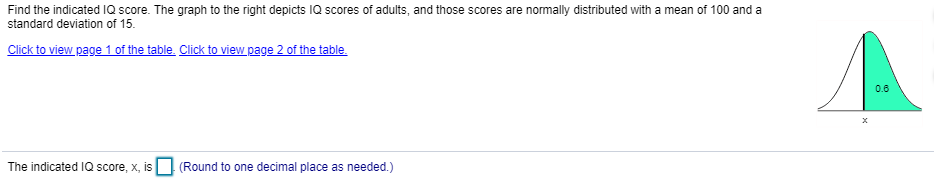# Find the indicated IQ score. The graph to the right depicts IQ scores of adults, and those scores are normally distributed with a mean of 100 and astandard deviation of 15.0.6The indicated la score, x, is。(Round to one decimal place as needed )

Questionhelp_outlineImage TranscriptioncloseFind the indicated IQ score. The graph to the right depicts IQ scores of adults, and those scores are normally distributed with a mean of 100 and a standard deviation of 15. 0.6 The indicated la score, x, is。(Round to one decimal place as needed ) fullscreen
check_circle

Step 1

The z-score:

The z-score of a normally distributed random variable, X, with mean μ and standard deviation σ, at the point x, is given as:

Step 2

Calculation:

The normally distributed IQ score, X has mean μ = 100 and standard deviation σ = 15.

According to the given graph, the probability of X, above the value x, is 0.6; that is, P (X > x) = 0.6....

### Want to see the full answer?

See Solution

#### Want to see this answer and more?

Solutions are written by subject experts who are available 24/7. Questions are typically answered within 1 hour.*

See Solution
*Response times may vary by subject and question.
Tagged in

### Data